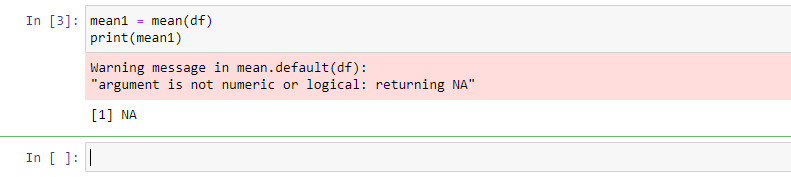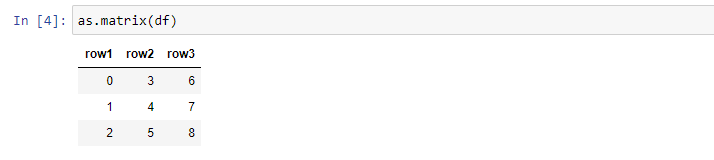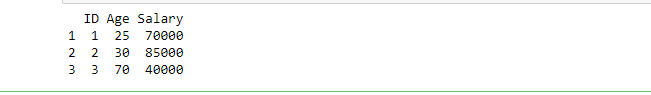Related Articles

# How to find the mean of all values in an R data frame?

• Last Updated : 30 May, 2021

In this article, we are going to find the mean of the values of a dataframe in R with the use of mean() function.

Syntax:

mean(dataframe)

### Creating a Dataframe

A dataframe can be created with the use of data.frame() function that is pre-defined in the R library. This function accepts the elements and the number of rows and columns that are required for the dataframe to be created.

Following is an R Program for the creation of dataframe:

## R

 `# R Program to create a dataframe`` ` `# Creating a Data Frame ``df<-``data.frame``(row1 = 0:2, row2 = 3:5, row3 = 6:8) ``print``(df)`

Output:

```  row1 row2 row3
1    0    3    6
2    1    4    7
3    2    5    8```

### Computing Mean of the Dataframe

R language provides an in-built function mean() to compute the mean of a dataframe. Following is an R program for the implementation of mean().

## R

 `mean1 = ``mean``(df)``print``(mean1)`

Output:Here in the above code, a warning message is displayed, which returns NA because the dataframe is not in a numeric form. There is a need to convert it into matrix form to compute the mean of the dataframe. R provides an inbuilt as.matrix() function to convert a dataframe to a matrix.

## R

 `# Converting dataframe to matrixa``as.matrix``(df)`

Output:Now, to compute mean of this matrix created from a dataframe, use the mean function on the matrix.

## R

 `# Finding mean of the dataframe`` ` `# Using mean() function``mean``(``as.matrix``(df))`

Output:

`4`

Example 2:

## R

 `# R program to illustrate dataframe ``Roll_num = ``c``(01, 02, 03)``Age = ``c``(22, 25, 45)``Marks = ``c``(70, 80, 90)``   ` `# To create dataframe use data.frame command and ``# then pass each of the vectors  ``# we have created as arguments ``# to the function data.frame() ``df = ``data.frame``(Roll_num, Age, Marks) ``   ` `print``(df)`

Output:## R

 `# Computing mean of the above dataframe`` ` `# Using the mean() function``mean``(``as.matrix``(df))`

Output:

`37.5555555555556`

Example 3:

## R

 `# R program to illustrate dataframe ``ID = ``c``(01, 02, 03)``Age = ``c``(25, 30, 70)``Salary = ``c``(70000, 85000, 40000)``   ` `# To create dataframe use data.frame command and ``# then pass each of the vectors  ``# we have created as arguments ``# to the function data.frame() ``df = ``data.frame``(ID, Age, Salary) ``   ` `print``(df)`

Output:## R

 `# Computing mean of the dataframe`` ` `# Using mean() function``mean``(``as.matrix``(df))`

Output:

`21681.2222222222`

My Personal Notes arrow_drop_up